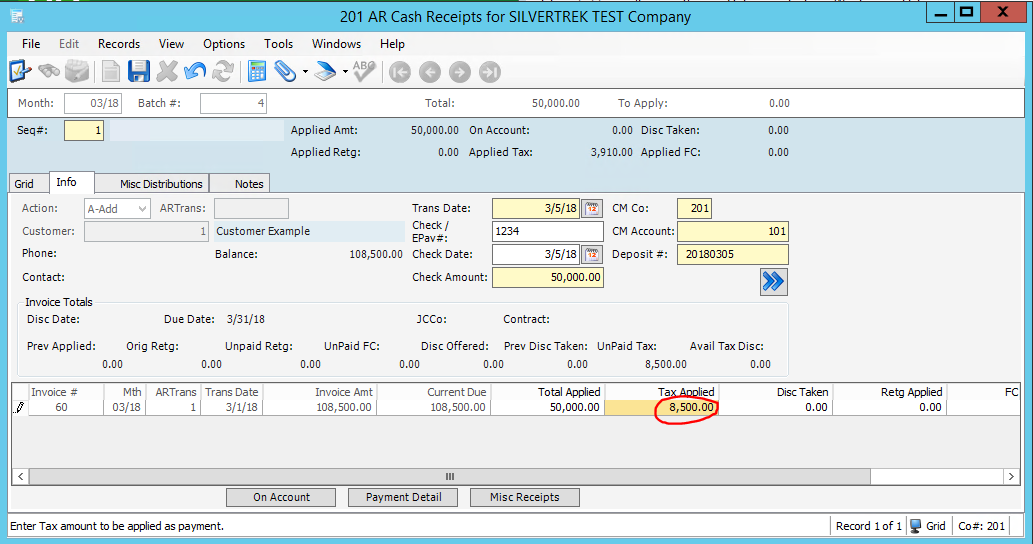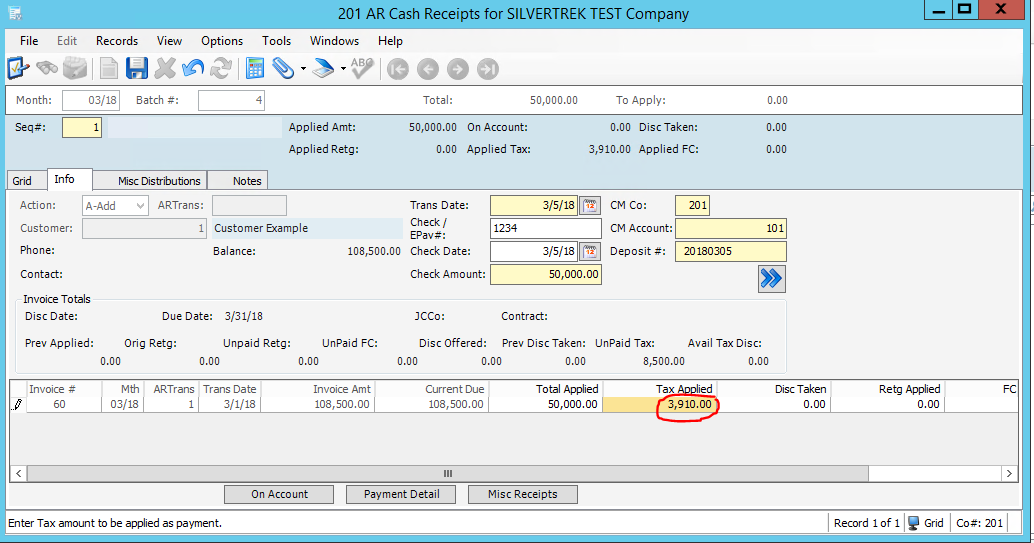# How to record partial AR receipts that have sales tax.

1. In Vista, select "Accounts Receivable"

2. In the "Programs" folder, select " AR Cash Receipts"

3. After selecting the appropriate month, fill in the "AR Cash Receipt" with the appropriate Customer and payment information.

4. Type the check amount into "Total Applied". After entering the total check amount, you can see that it has applied the full invoice tax amount, instead of the partial payment tax amount.5. Using a calculator, divide the partial payment by the total invoice amount, including tax (per example 50,000/108,500). The quotient amount of this equation is the percentage of tax that should be paid towards the partial payment. (.46 – 46%)

6. Using a calculator, take the total invoice tax amount and multiply by the percentage of tax that should be paid per the partial payment amount (per example: 8,500*.46). The product of this equation is the tax amount that should be applied for the partial payment.

7. Enter the tax amount per partial payment to the “Tax Applied” portion.8. Save Record

9. Click “File” and then “Process Batch”